+
Absolute Value Inequalities
Solving Linear Equations and Inequalities
0
of 0 possible points

# Absolute Value Inequalities

Author: Sophia Tutorial
##### Description:

Solve an absolute value inequality.

(more)

Sophia’s self-paced online courses are a great way to save time and money as you earn credits eligible for transfer to many different colleges and universities.*

No credit card required

46 Sophia partners guarantee credit transfer.

299 Institutions have accepted or given pre-approval for credit transfer.

* The American Council on Education's College Credit Recommendation Service (ACE Credit®) has evaluated and recommended college credit for 33 of Sophia’s online courses. Many different colleges and universities consider ACE CREDIT recommendations in determining the applicability to their course and degree programs.

Tutorial

what's covered
1. "Less Than" Absolute Value Inequalities
2. "Greater Than" Absolute Value Inequalities
3. Solving Absolute Value Inequalities
4. No Solutions or All Real Solutions

# 1. "Less Than" Absolute Value Inequalities

When an inequality has an absolute value we will have to remove the absolute value in order to graph the solution or give interval notation. The way we remove the absolute value depends on the direction of the inequality symbol.

Consider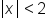.

Absolute value is defined as distance from zero. Another way to read this inequality would be the distance from zero is less than 2. So on a number line we will shade all points that are less than 2 units away from zero.This graph looks just like the graphs of the three part compound inequalities! When the absolute value is less than a number we will remove the absolute value by changing the problem to a three part inequality, with the negative value on the left and the positive value on the right. Sobecomes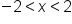, as the graph above illustrates.

big idea
If the absolute value inequality is "less than" or "less than or equal to", we can write this as:
inequality: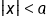rewrite as: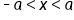The inequality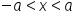is a type of "AND" compound inequality.
formula

Absolute Value Inequalities - Less Than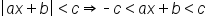# 2. "Greater Than" Absolute Value Inequalities

Consider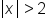.

Absolute value is defined as distance from zero. Another way to read this inequality would be the distance from zero is greater than 2. So on the number line we shade all points that are more than 2 units away from zero.This graph looks just like the graphs of the OR compound inequalities! When the absolute value is greater than a number we will remove the absolute value by changing the problem to an OR inequality, the first inequality looking just like the problem with no absolute value, the second flipping the inequality symbol and changing the value to a negative. Sobecomes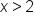or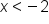, as the graph above illustrates.

big idea
If the absolute value inequality is "greater than" or "greater than or equal to", we can write this as:
inequality: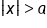rewrite as: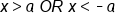This inequality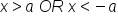is a type of "OR" compound inequality.
formula

Absolute Value Inequalities - Greater Than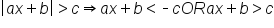# 3. Solving Absolute Value Inequalities

We can solve absolute value inequalities much like we solved absolute value equations by following these steps.

step by step

1. Make sure the absolute value is isolated on one side.
2. Remove the absolute value by either making a three-part inequality if the absolute value is less than a number, or making an OR inequality if the absolute value is greater than a number.
3. Solve the inequality.
hint
Remember, if we multiply or divide by a negative the inequality symbol will switch directions!

EXAMPLE

Solve, graph, and give interval notation for the solution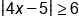Absolute value is greater, use OR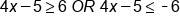Solve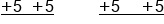Add 5 to both sides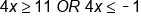Divide both sides by 4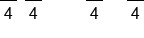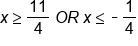Graph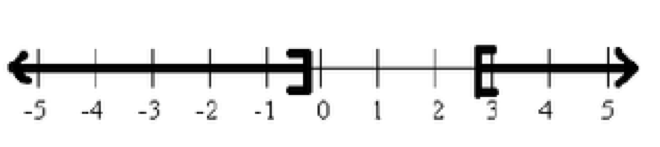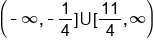Interval notation

hint
For all absolute value inequalities, we can also express our answers in interval notation which is done the same way it is done for standard compound inequalities.

EXAMPLE

Solve, graph, and give interval notation for the solution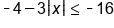Add 4 to both sides to isolate the absolute value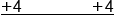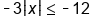Divide both sides by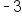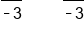Dividing by a negative switches the symbol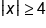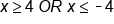Absolute value is greater, use OR; Graph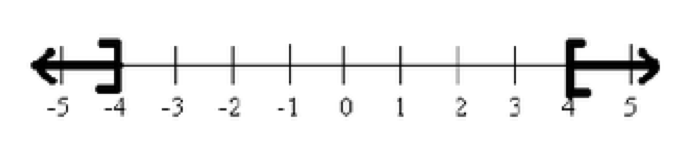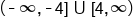Interval notation

hint
In the previous example, we cannot combine −4 and−3 because they are not like terms, the − 3 has an absolute value attached. So we must first clear the − 4 by adding 4, then divide by −3. The next example is similar.

EXAMPLE

Solve, graph, and give interval notation for the solution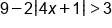Subtract 9 from both sides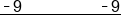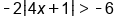Divide both sides by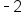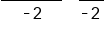Dividing by negative switches the symbol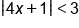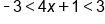Absolute value is less, use three part; Solve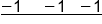Subtract 1 from all three parts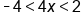Divide all three parts by 4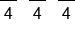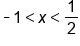Graph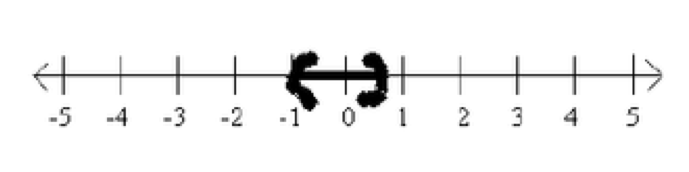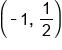Interval Notation

hint
In the previous example, we cannot distribute the − 2 into the absolute value. We can never distribute or combine things outside the absolute value with what is inside the absolute value. Our only way to solve is to first isolate the absolute value by clearing the values around it, then either make a compound inequality (either a three-part inequality or an OR inequality) to solve.

# 4. No Solutions or All Real Solutions

It is important to remember as we are solving these equations, the absolute value is always positive. There are cases where there is no solution to the inequality or all real numbers are the solution:

• No Solutions: If we end up with an absolute value is less than a negative number, then we will have no solution because absolute value will always be positive, greater than a negative.
• All Real Solutions: If the absolute value is greater than a negative, this will always happen. Here the answer will be all real numbers.
Solve, graph, and give interval notation for the solution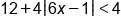Subtract 12 from both sides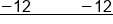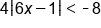Divide both sides by 4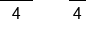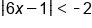Absolute value can't be less than a negative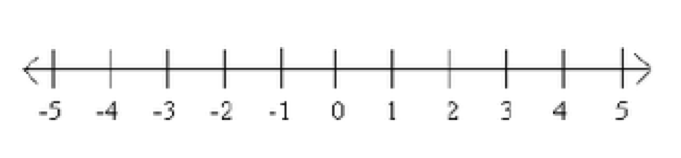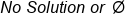Interval Notation

Solve, graph, and give interval notation for the solution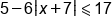Subtract 5 from both sides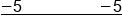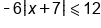Divide both sides by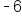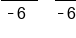Dividing by a negative flips the symbol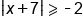Absolute value always greater than negative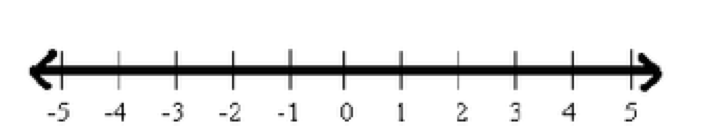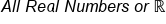summary
"Less than" absolute value inequalities can be rewritten as "AND" compound inequalities, where our expression and our absolute value sign is bound between the negative and positive values of this quantity. "Greater than" absolute value inequalities can be written as "OR" compound inequalities, where your expression inside your absolute value sign is going to be less than the negative of this quantity, or it's going to be greater than the positive value of this quantity. When solving absolute value inequalities, remember to first isolate the absolute value, then remove the absolute value by either making a three-part inequality if the absolute value is less than a number, or making an OR inequality if the absolute value is greater than a number. There are also some instances where there is no solutions or all real solutions.

Source: Adapted from "Beginning and Intermediate Algebra" by Tyler Wallace, an open source textbook available at: http://wallace.ccfaculty.org/book/book.html

Formulas to Know
Absolute Value Inequalities - Greater ThanAbsolute Value Inequalities - Less ThanRating